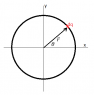# Electric dipole moment for a uniformly charged ring

• FallenLeibniz
In summary: So##p_x = \int_{- \pi/2}^{\pi/2}{a \cos \theta \lambda a d\theta}####p_x = \lambda a^2 \int_{- \pi/2}^{\pi/2}{\cos \theta d\theta}####p_x = \lambda a^2 \left( \sin \theta \right)_{-\pi/2}^{\pi/2}####p_x = \lambda a^2 \left( 1 - (-1) \right)####p_x = 2 \lambda a^2##Similarly, ##p_y## gives the same answer. ##p_z##

## Homework Statement

Text description: Let V(z) be the potential of a ring of charge on the axis of symmetry at
distance z from the center. Obtain the first two non-vanishing terms of the multipole expansion
for V(z) with z>>a where a is the radius of the ring. Can you see by symmetry that the dipole moment is
zero?

My issue: While I was able to do the monopole term just fine. The problem is with the dipole
moment term. My work (outlined below) keeps giving me a positive term, however intuition
and the geometry of the problem (as well at the text's statement of the problem)
dictate that the dipole moment is zero. Can anyone point out where I went wrong in my work?

2. Homework Equations
a)Definition of the Electric Dipole Moment for a continuous body
##\vec p(\vec{x'})=\int \vec{x'} \lambda {dx'}##

b)My Setup
##\vec p(\vec{x'})=\lambda a^2 \int_{0}^{2\pi}\hat{s}{d\phi'}##[/B]

## The Attempt at a Solution

The following is the result I have gotten to thus far.
[/B]
##\vec p(\vec{x'})={2\pi}\lambda a^2\hat{s}##

##\hat{s}## is not a constant vector in the integration.

See, I am aware of that, but I am not quite sure how to decompose it such that all of the source point vectors cancel out when you integrate. Do I have to switch over to Cartesian neccesarily?

I would switch to Cartesian coordinates and show that each Cartesian component of the dipole moment vector p is zero.

I tried that, but so far I've been stumped in that way. However, another way came to mind. It gives the right answer and would like your opinion. If you orient the ring such that the diameter is collinear with the z-axis, the differential length along the curve will equal to ##a{d\theta}##. If you integrate over the whole ring, you will first have to integrate from 0 to ##\pi## then you would integrate from ##\pi## to 0. The result does end up giving zero (as stated before) and seems plausible enough, but I would be grateful for any second opinions from anyone. :)

Implicit in my above post is the fact that I have switched to Spherical Coordinates to work the problem.

It might be confusing to use ##\vec{x}'## as the position vector of an element of charge. Maybe use ##\vec{r}## instead. Then ##\vec{p} = \int{\vec{r} dq}##. The x-component would be ##p_x = \int{x dq}##. Write ##xdq## in terms of ##a##, ##\theta## and ##d\theta##. See what you get.

#### Attachments

•ring.png
2.1 KB · Views: 1,164
I am not quite sure I understand why I would have to solve for the x-component after going to Spherical. Are you saying try to convert what I have in Spherical to Cartesian just for the purposes of verifying my results?

The way I suggested to do it is just a way that seems natural to me. The integrals for ##p_x## and ##p_y## are very simple to evaluate. ##p_z## is trivial. (I'm taking the ring to lie in the xy plane with the z-axis running along the axis of the ring.)

My reasoning for post 5 was the following:

Orient the ring of charge such that the diameter is collinear with the Z-axis.

Since, in Spherical Coordinates ##\theta## is defined as the angle from the positive z-axis, the ring is divided into two parts. Thus:

##\vec{p}=\int_{ring} \vec{r'}\lambda dC'##

The result when I evaluate does give 0. However, it is a bit hackish. I am working on trying the approach you suggested now. It's made it a bit easier as well the fact that I realized that ##a=({x'}^2+{y'}^2)^{1/2}##. I believe that it will cut down on the work a bit. Thank you.

p.s.
Once I have the solution, should I post back to say that I got it?

Last edited:
FallenLeibniz said: